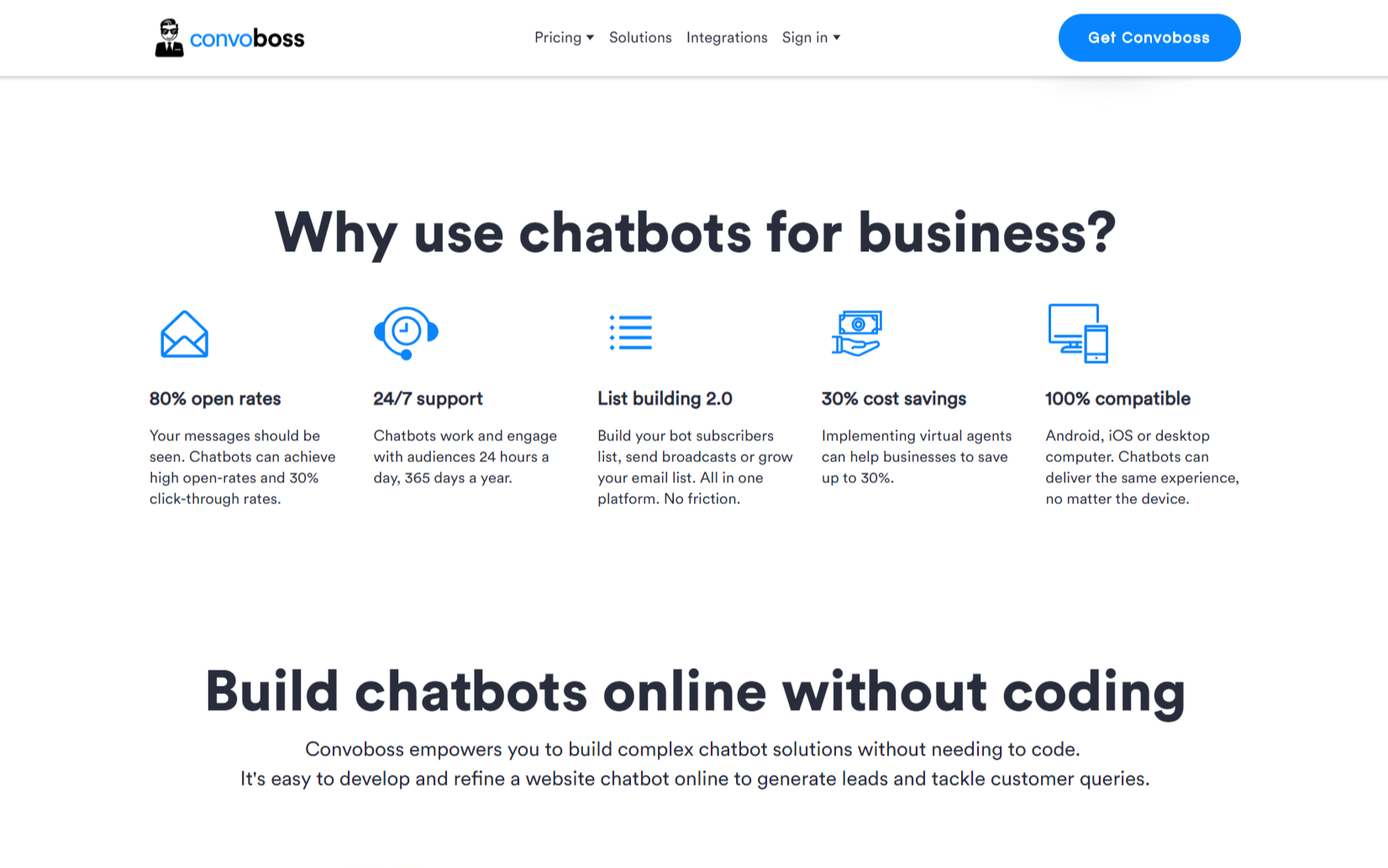# Percentage Calculator Chatbot

This free chatbot calculator solves two percentage calculations: X / Y = P%, and P% * X = Y

Chatbot Calculator — Percentages

Convoboss makes chatbot calculators worthy
of conversation